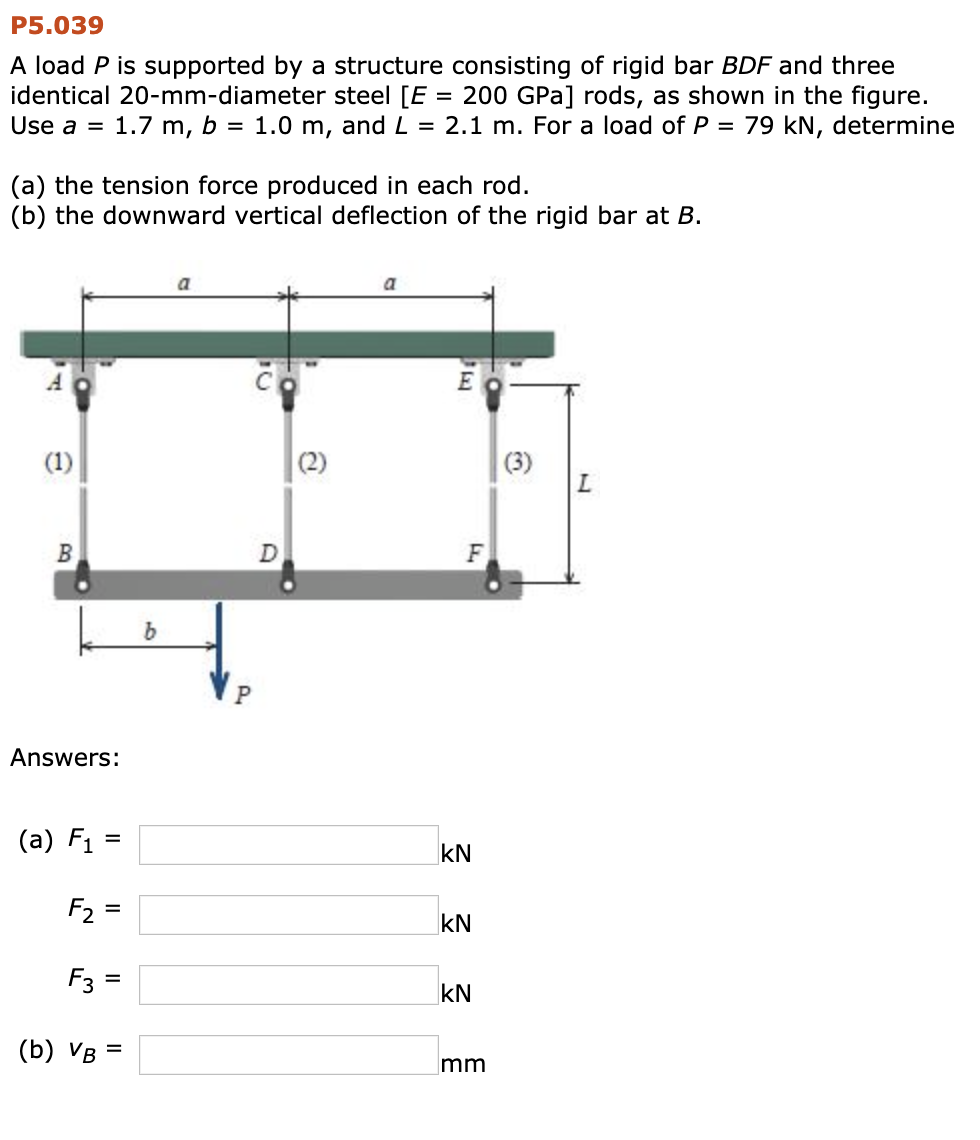# Question P5.039 A load P is supported by a structure consisting of rigid bar BDF and three identical 20-mm-diameter steel [E = 200 GPa] rods, as shown in the figure. Use a = 1.7 m, b = 1.0 m, and L = 2.1 m. For a load of P = 79 kN, determine (a) the tension force produced in each rod. (b) the downward vertical deflection of the rigid bar at B. P5.039 A load P is supported by a structure consisting of rigid bar BDF and three identical 20-mm-diameter steel [E = 200 GPa) rods, as shown in the figure. Use a = 1.7 m, b = 1.0 m, and L = 2.1 m. For a load of P = 79 kN, determine (a) the tension force produced in each rod. (b) the downward vertical deflection of the rigid bar at B. Answers: (a) F1 = F2= (b) vb =DMES2W The Asker · Mechanical Engineering

P5.039

A load P is supported by a structure consisting of rigid bar BDF and three identical 20-mm-diameter steel [E = 200 GPa] rods, as shown in the figure. Use a = 1.7 m, b = 1.0 m, and L = 2.1 m. For a load of P = 79 kN, determine

(a) the tension force produced in each rod.
(b) the downward vertical deflection of the rigid bar at B.Transcribed Image Text: P5.039 A load P is supported by a structure consisting of rigid bar BDF and three identical 20-mm-diameter steel [E = 200 GPa) rods, as shown in the figure. Use a = 1.7 m, b = 1.0 m, and L = 2.1 m. For a load of P = 79 kN, determine (a) the tension force produced in each rod. (b) the downward vertical deflection of the rigid bar at B. Answers: (a) F1 = F2= (b) vb =
More
Transcribed Image Text: P5.039 A load P is supported by a structure consisting of rigid bar BDF and three identical 20-mm-diameter steel [E = 200 GPa) rods, as shown in the figure. Use a = 1.7 m, b = 1.0 m, and L = 2.1 m. For a load of P = 79 kN, determine (a) the tension force produced in each rod. (b) the downward vertical deflection of the rigid bar at B. Answers: (a) F1 = F2= (b) vb =